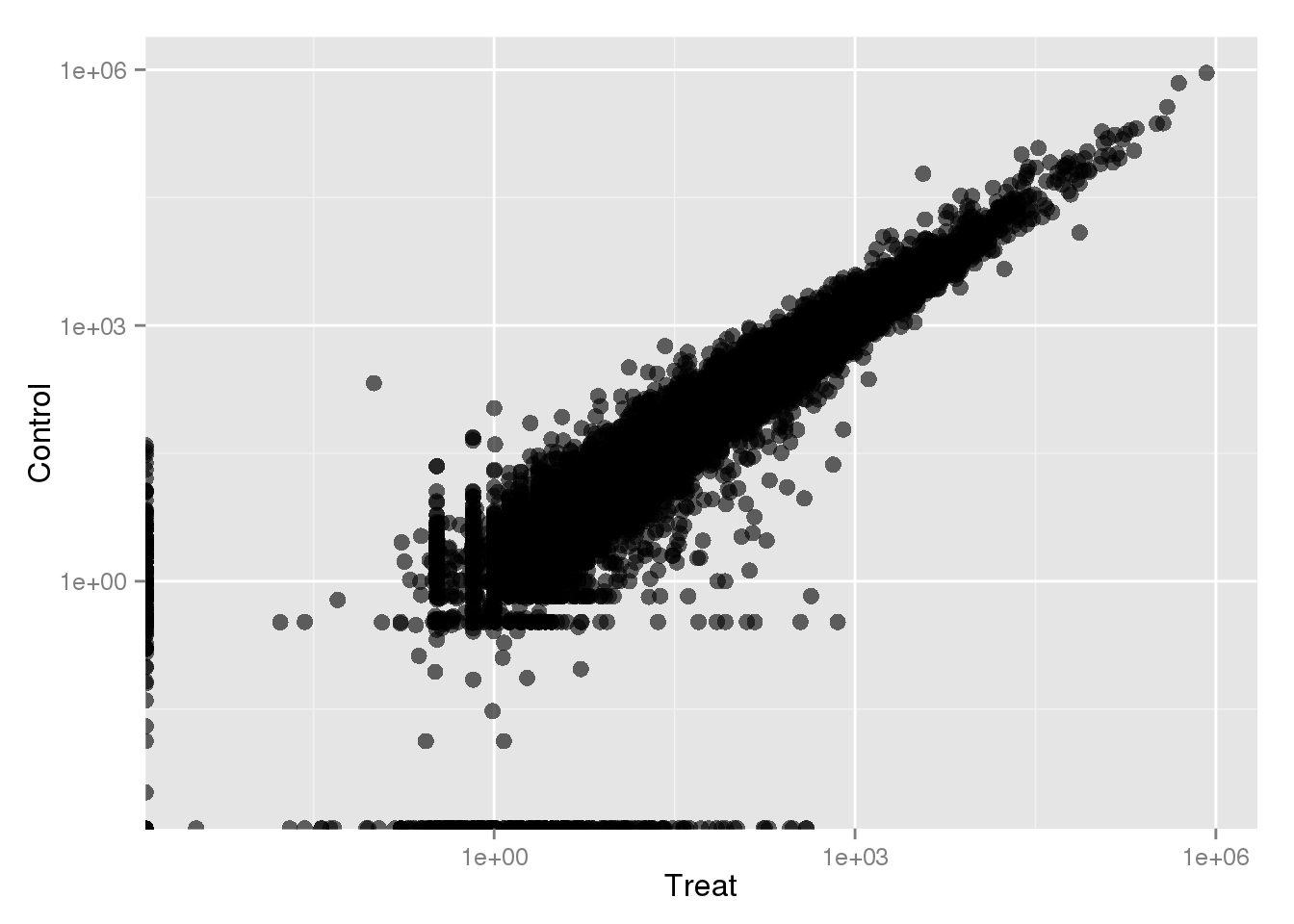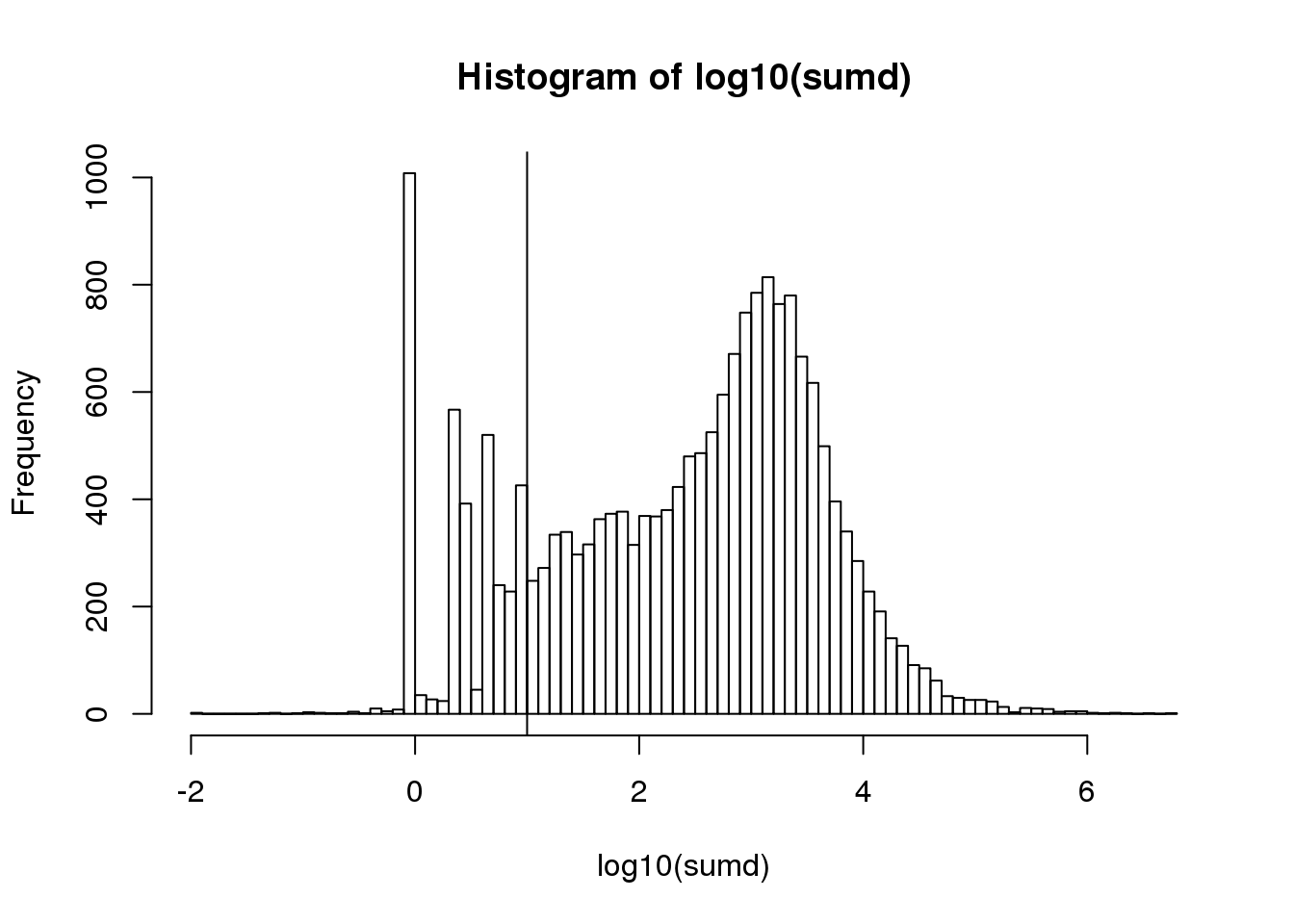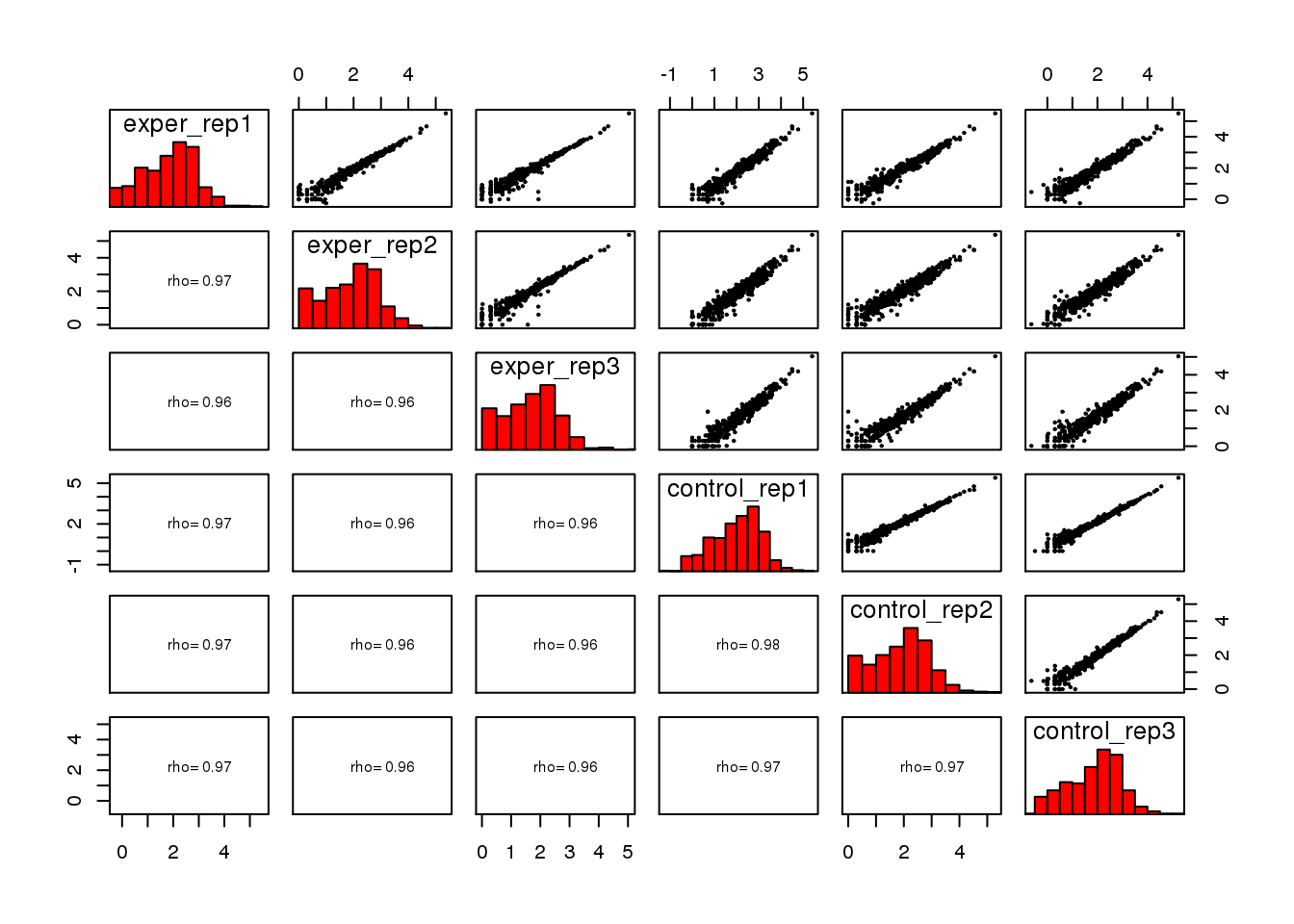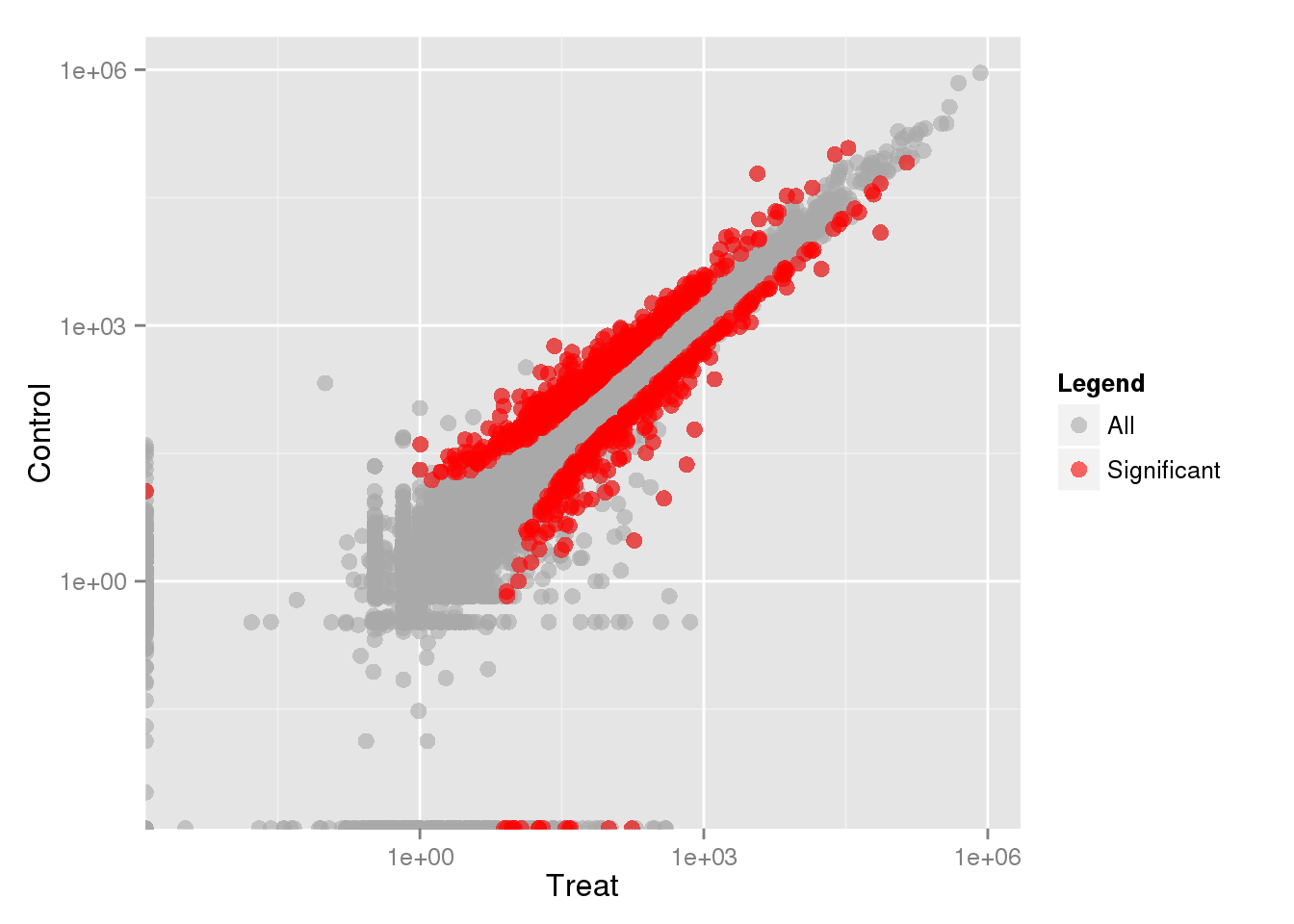s class.R
``````#Tip: To run any line in RStudio you can use Ctrl+Enter or Command+Enter.
#To use any package in R, we need to load its library first.To run DESeq2
#you just need to type the following.
library("DESeq2")``````
``````## Loading required package: S4Vectors
##
## Attaching package: 'BiocGenerics'
##
## The following objects are masked from 'package:parallel':
##
##     clusterApply, clusterApplyLB, clusterCall, clusterEvalQ,
##     clusterExport, clusterMap, parApply, parCapply, parLapply,
##     parLapplyLB, parRapply, parSapply, parSapplyLB
##
## The following objects are masked from 'package:stats':
##
##
## The following objects are masked from 'package:base':
##
##     anyDuplicated, append, as.data.frame, as.vector, cbind,
##     colnames, do.call, duplicated, eval, evalq, Filter, Find, get,
##     grep, grepl, intersect, is.unsorted, lapply, lengths, Map,
##     mapply, match, mget, order, paste, pmax, pmax.int, pmin,
##     pmin.int, Position, rank, rbind, Reduce, rownames, sapply,
##     setdiff, sort, table, tapply, union, unique, unlist, unsplit
##
## Welcome to Bioconductor
##
##     Vignettes contain introductory material; view with
##     'browseVignettes()'. To cite Bioconductor, see
##     'citation("Biobase")', and for packages 'citation("pkgname")'.
##
``source("https://bioinfo.umassmed.edu/content/pub/funcs.R")``
``````##
## Attaching package: 'gplots'
##
## The following object is masked from 'package:IRanges':
##
##     space
##
## The following object is masked from 'package:stats':
##
##     lowess``````
``````#1. Reading the data.
# a single table. Here we prepared another table using whole reads
# (not only using reduced reads) to give you an idea about the complete
# picture of DESeq analysis.
url <-  "https://galaxyweb.umassmed.edu/pub/class/data.tsv"
#install a package
install.packages("RCurl")
library(RCurl)
file <- textConnection(getURL(url))

#file <- "/project/umw_biocore/class/data.tsv"

#1. Read the data with row.names. We have the gene names in the
# first column in this table. When we set row.names=1 It will remove
# the first column from the data and put them to the row name section.

#2. Creating the data structure for DESeq Analysis, we need to select
# the columns that we are going to use in the analysis. Here we are
# going to use experiment and control data that will be 6 columns.
# Here in this step make sure to include the columns you want to use in
columns <- c("exper_rep1","exper_rep2","exper_rep3",
"control_rep1","control_rep2","control_rep3")

data <- data.frame(rsem[, columns])

#3. Scatter plots are a key approach to assess differences between conditions.
# We plot the average expression value (counts or TPMs for example) of
# two conditions.First calculate the average reads per gene. To find the
# average we sum experiments per gene and divide it to the number of replicas

# In this case we will divide the sum to 3. We will calculate the average for
# control libraries too. After that, we are ready to merge  the average values
# from experiment and control to create a two column  data structure using the
# cbind function that merges tables.

avgall<-cbind(rowSums(data[c("exper_rep1","exper_rep2","exper_rep3")])/3,
rowSums(data[c("control_rep1","control_rep2","control_rep3")])/3)

# We can also change the column names using colnames function.

colnames(avgall)<-c("Treat", "Control")

#4.There are several packages in R that can be used to
# generate scatter plots. The same plot can be generated using
# the ggplot package.

gdat<-data.frame(avgall)
ggplot() +
geom_point(data=gdat, aes_string(x="Treat", y="Control"),
colour="black", alpha=6/10, size=3) +
scale_x_log10() +scale_y_log10()````````````#5. In Eukaryotes only a subset of all genes are expressed in
# a given cell. Expression is therefore a bimodal distribution,
# with non-expressed genes having counts that result from experimental
# and biological noise. It is important to filter out the genes
# that are not expressed before doing differential gene expression.

# You can decide which cutoff separates expressed vs non-expressed
# genes by looking your histogram we created.

# In our case a total sum of 10 counts separates well expressed
# from non-expressed genes

sumd <- rowSums(data)
hist(log10(sumd), breaks=100)
abline(v=1)````````````#To make all to all scater plot, use the function below
all2all(data)````````````######################################
## LETS START DESeq ANALYSIS
######################################
#
#6. The goal of Differential gene expression analysis is to find
# genes or transcripts whose difference in expression, when accounting
# for the variance within condition, is higher than expected by chance.

# The first step is to indicate the condition that each column (experiment)
# in the table represent.

# Here we define the correspondence between columns and conditions.
# Make sure the order of the columns matches to your table.

columns``````
``````##  "exper_rep1"   "exper_rep2"   "exper_rep3"   "control_rep1"
##  "control_rep2" "control_rep3"``````
``````conds <- factor( c("Control","Control", "Control",
"Treat", "Treat","Treat") )

# DESeq will compute the probability that a gene is differentially
# expressed (DE) for ALL genes in the table. It outputs both a
# nominal and a multiple hypothesis corrected p-value (padj).
# Because we are testing DE for over 20,000 genes, we do need to correct
# for multiple hypothesis testing. To find genes that are significantly
# DE, we select the ones has lower padj values and higher fold changes and
# visualize them on our scatter plot with different  color.
# padj values are corrected p-values which are multiplied by the number
# of comparisons. Here we are going to use 0.01 for padj value
# and > 1 log2foldchange.  (1/2 < foldChange < 2)

de_res <- runDESeq(data, columns, conds,  padj=0.01, log2FoldChange=1, non_expressed_cutoff=10)``````
``````## estimating size factors
## estimating dispersions
## gene-wise dispersion estimates
## mean-dispersion relationship
## final dispersion estimates
## fitting model and testing``````
``````overlaid_data <- overlaySig(gdat, de_res\$res_selected)

#7. Make ggplot and overlay the significantly DE genes in the scatter plot

ggplot() +
geom_point(data=overlaid_data, aes_string(x="Treat", y="Control",
colour="Legend"), alpha=6/10, size=3) +
scale_colour_manual(values=c("All"="darkgrey","Significant"="red"))+
scale_x_log10() +scale_y_log10()````````````#8. The Second way to visualize it, we use MA plots.
# For MA Plot there is another builtin function that you can use.
plotMA(de_res\$res_detected,ylim=c(-2,2),main="DESeq2");``````